# Notes on Topology and Geometry

This collection of definitions and results is the product of a lengthy fishing trip for interesting and perhaps useful information. No pretense is claimed that it is in any way complete. Any errors are the sole responsibility of the fisherman, and will be cheerfully corrected as they are found.
Some of this content comes from work done trying to understand what I have found. As such, it represents the current state of my understanding (and ignorance) of the topic. This content, too, will be cheerfully amended as my understanding increases.
Some notation:
Rn denotes the open set of reals in n dimensions.
Sn denotes the n-dimensional sphere (S1 is a circle, S2 is the surface of a ball, etc.).
Bn denotes the n-dimensional ball (B1 is a line segment with end points, B2 is a disc, etc.).
In is the unit line segment, square, cube, etc.
Zn is the group of integers modulo n.

A group is a set of elements closed under an associative multiplication operator (a product is a member of the group), which possesses an identity element (I), such that every element has an inverse (G G-1 = I). The number of elements in the group is the order.

Q = G / H is the quotient group, such that for all elements g in G - H and h in H, g h g-1 is in H (h is conjugated by g and H is a normal subgroup), and H is the equivalency class of the identity element in Q.

O(n) is the group of rotations in n dimensions; SO(n) is the subgroup of O(n) which includes the identity. As with all special groups, its elements have unit determinant.

## On n-Manifolds

This section applies to spaces of arbitrary dimension.

#### Homotopy

• Two maps of Sk into M are said to be homotopically equivalent if they are continuously deformable into one another.

The set of equivalence classes of such maps forms a group πk(M), the kth homotopy group of M.
π1 is called the fundamental group.

π1 are isomorphic for homeomorphic manifolds.

• If X is the union of two manifolds M and N, and M ∩ N is simply connected, π1(X) is the free product of π1(M) and π1(N).
The free product is formed by taking all products of the elements of each group (where any two adjacent factors are from different groups), and reducing the product by removing any adjacent factors which are equivalent to the identity.
• π1(S3) = 0.

Every closed manifold homotopy equivalent to Sn is homeomorphic to Sn (the Poincare Conjecture).

A homotopy sphere is an n-manifold homotopically equivalent to Sn. An exotic sphere is a homotopy sphere which is not diffeomorphic to Sn. An "ordinary sphere" has a smooth structure inherited from its embedding into Rn+1. The following table gives the number of diffeomorphism classes of spheres in dimensions 1 through 15:

 1 2 3 4 5 6 7 8 9 10 11 12 13 14 15 1 1 1 ∞ ? 1 1 28 2 8 6 992 1 3 2 16256
(Averett, p. 7, 8, 10)
• π1(M) is non-abelian if M is a torus of genus > 1, or a connected sum of RP2.
The genus is the number of "handles". g = 1 for the surface of a donut.
The following illustrate the nonintuitive result that higher-dimensional spheres can be mapped onto lower-dimensional spheres:
πk(Sn) = πk(S2n-1) = πk-1(Sn-1).
πn+1(Sn) = πn+2(Sn) = Z2 for n > 2.
πk(S2) = πk(S3) for k > 2.
π3(S2) = Z.
πk(S2) = Z2 for k = 4, 5, 7 and 8.
π6(S2) = Z12.
π9(S2) = Z3.
π10(S2) = Z15.
(Hu, p. 58, 153, 325, 328, 329, 332)
• A manifold is simply connected if π1 = 0.
• The universal cover of a manifold M is locally homeomorphic to M but is simply connected, with discrete fibers over M. If the cover is locally pathwise connected, the fiber group is isomorphic to π1(M).
A fiber bundle is a total space E which locally is a Cartesian product of the fiber space F and the base space M, with a projection E → M.

A connection is a field transverse to the fibers which defines motion among fibers relative to motion in the base manifold; it is the same dimension as the base manifold. Except in the trivial (flat) case, it can only be defined within a local coordinate patch.

SO(3) is topologically RP3, with π1 = Z2, and universal cover S3.
π1(SO(n)) = Z2.
The proper Lorentz Group is topologically RP3R3, with universal cover SL(2,C).
For k > 1, πk(M) = πk(E) if E is the universal cover of M. (Greenberg, p. 24, 30, 35)

If M is a closed surface other than S2 or RP2, π1(M) is infinite and the universal cover is R2. (Hu, p. 96)

• If G is a simply connected group and H is a discrete normal subgroup, then in the neighborhood of the identity, π1(G/H) is isomorphic to H (and is abelian). (Greenberg, p. 18)
• For any finitely presented group G, for n > 3 there is an n-manifold such that π1(M) = G. (Averett, p. 7)
• Every connected non-orientable manifold has a 2-fold connected orientable cover.
Every connected manifold whose π1 does not contain a subgroup of index 2 is orientable.
The index of a subgroup H is the number of elements x hi in G where hi is in H and x is not in H.
Of Sn, T2 (of arbitrary genus), RPn, CPn and HPn, only RPn for n even is not orientable. (Greenberg, p. 162, 168)
• If G is a Lie group acting transitively, analytically and with compact stabilizers on a simply connected space X and M is a closed differentiable manifold, (G, X) structures on M (up to diffeomorphism) are in 1:1 correspondence with conjugacy classes of discrete subgroups of G that are isomorphic to π1(M) and act freely on X with quotient M.
The tangent space of a Lie group at the identity has the structure of a Lie algebra: a vector space equipped with a commutator [x,y] = - [y,x] which statisfies the Jacobi identity [[x,y],z] + [[y,z],x] + [[z,x],y] = 0.

The stabilizers of a group are those elements which leave fixed points.

A (G, X) structure on a space X is a maxmial coordinate chart such that the coordinate systems in every overlapping patch are related by elements of the group G; a diffeomorphism class of metrics on M.

The elements gi form a conjugacy class if for all elements x in G, x-1 gi x is one of the gi.

Diffeomorphism classes of closed n-Euclidean manifolds are in 1:1 correspondence, via their fundamental groups, with torsion-free groups containing a subgroup of finite index isomorphic to Z n.
A torsion element x satisfies xm = I for some integer m.
(Thurston, p. 157, 167, 222)
• If φ is a homotopy equivalence between closed, connected, orientable, hyperbolic n-manifolds (n > 2), then φ is homotopic to an isometry. This is Mostow's rigidity theorem. (Ratcliffe, p. 596)
For M hyperbolic and k > 1, πk(M) = 0. (Marden, section 5.2)

#### Homology

• Let M be a smooth connected manifold. A p-chain is Σi ci Ni, where the Ni are smooth, oriented p-submanifolds of M. (Eguchi, Gilkey and Hanson, p. 233)
• A cycle is a chain with no boundary.
• The pth homology group is Hp(M;G) = Qp / Bp, where G is one of R, C, Z or Z2, Qp is the set of p-cycles and Bp is the set of p-boundaries in M (a subset of Qp). It is the set of equivalence classes of cycles which differ only by boundaries; equivalently, the set of equivalence classes of cycles which are not boundaries of a sub-manifold Np+1.

Hp(M;G) = 0 for p > n = dim(M).
If M is connected, H0(M;G) = G.
If M is orientable, Hn(M;G) = G.
If M is orientable and G is R, C or Z2, Hp(M;G) = Hn-p(M;G) (Poincare Duality). (Eguchi, Gilkey and Hanson, p. 233)

Hp(Sn) is isomorphic to Hp-1(Sn-1) for p > 1.
Hp(RPn) = G2 (submodule annihilated by multiplication by 2) for p even > 1; G / 2 for p odd > 0, < n; G for p = 0 or n odd. (Greenberg, p. 83, 121)

H1 = π1 / [π1, π1]. (Bott and Tu, p. 225)

[G, G] is the commutator subgroup of G: the subgroup of G generated by all of the commutators of G. It is the smallest normal subgroup of G such that G / [G, G] is abelian.
If E is the universal cover of M, π2(M) is isomorphic to H2(E).
If πk(M) = 0 for k < p, πp(M) is isomorphic to Hp(M). (Hurewicz Theorem) (Hu, p. 154, 167)
• bp is the pth Betti number, equal to dim(Hp(M;R)). It is a topological invariant.

b0 = the number of connected components.

bp = 0 (0 < p < n) for a compact, orientable manifold of positive constant curvature (M is a homology sphere).
bp <= (n p) for a compact, orientable, locally flat manifold. Tn saturates that bound.
bp = 0 (0 < p < n) for a compact, orientable conformally flat manifold of positive definite Ricci curvature. (Goldberg, p. 89, 118; see also pp. 91, 92, 131)

b1 = b2 = 0 for a compact semi-simple Lie group (metric is non-degenerate). b3 = 1 for a compact simple Lie group. (Goldberg, p. 140, 145; see also p. 144)

bp = 0 for RPn except for b0 = 1, and bn = 1 if n is odd.
If M is a compact submanifold of Rn (n > 1) with k connected components, bn-1(Rn - M) = k. (Greenberg, p. 129, 167)

• The Euler Characteristic of a manifold without boundary M is χ(M) = Σp (-1)p bp.

An n-manifold M is equivalent to the product of a 1-manifold and an (n-1)-manifold iff χ(M) = 0. (Thurston, p. 115)

• If M = M1 ⊗ M2, Hk(M;R) = Σp + q = k Hp(M;R) ⊗ Hq(M;R) (Kunneth formula).

Further, χ(M) = χ(M1) χ(M2). (Eguchi, Gilkey and Hanson, p. 238)

If M and N are connected n-manifolds, χ(M # N) = χ(M) + χ(N), - 2 if n is even. (Greenberg, p. 131)

• If K is an n-fold cover of M, χ(K) = n χ(M). (Scott, p. 426)
• Hp(M;Z) is isomorphic to the cobordism group of M for p < 4. (Kauffman, p. 319)

#### Characteristic Classes

• The Stiefel-Whitney classes of a real vector bundle are the Z2 cohomology classes.
H1(M;Z2) is zero iff M is orientable.
If H2(M;Z2) is nonzero, it is not possible to consistently define spinors on M. (
Eguchi, Gilkey and Hanson, p. 316)

M is a boundary of a smooth compact manifold iff the Stiefel-Whitney numbers of M are all zero.

Characteristic numbers are topologically invariant integrals of elements of Hp(M;G) over p-chains.
Two smooth closed n-manifolds belong to the same cobordism class iff their Stiefel-Whitney numbers are all equal. (Milnor and Stasheff, p. 52, 53)
• The Pontrjagin class of a real vector bundle of dimension > 3 is
Det(I - Ω / 2π) = 1 + p4 + p8 + ...
where Ω is the O(k) curvature of the bundle. (Eguchi, Gilkey and Hanson, p. 311)

If some Pontrjagin number of M4 k is nonzero, M cannot possess any orientation-reversing diffeomorphism, and M cannot be the boundary of a smooth compact oriented manifold with boundary.

M is an oriented boundary iff all Pontrjagin numbers and Stiefel-Whitney numbers are zero. (Milnor and Stasheff, p. 186, 217)

• The Euler class of a real even-dimensional orientable vector bundle is the sum of the traces of the wedge products of the SO(k) curvature 2-form. If the manifold has boundary, the integral over M which defines the Euler characteristic must be corrected by the Chern-Simons term; ie., in four dimensions:
tr A ^ dA + (2/3) A ^ A ^ A
where A is the connection one-form. (Eguchi, Gilkey and Hanson, p. 313, 350)
• If κ (= 1, 0, -1) is the sectional curvature of a closed manifold Mn (n even),
χ(M) = 2 κn/2 Vol(M) / Vol(Sn)
This is the Gauss-Bonnet theorem.

If M is also orientable and hyperbolic, χ(M) is even. If n is even and M is hyperbolic and of finite volume,

Vol(M) = (-1)n/2 χ(M) Area(Sn-1) / 2
(Marden, section 4.10)
• The Lefschetz fixed point theorem states that the Euler characteristic is the number of fixed points of an isometry on M. It is also equal to the number of zeroes of the Killing vector field defined by f.

If f is not an isometry, the Euler characteristic is the signed sum of the number of zeroes, where the sign is determined by whether or not the diffeomorphism preserves the orientation. (Eguchi, Gilkey and Hanson, p. 335)

The sign here comes from the index, which is +1 for a source or a sink, and -1 for a saddle. (Thurston, p. 23)
• The characteristic classes are all topological invariants. They must vanish if a global section is to exist on the bundle.
A bundle section is a continuous map from the base space to the total space. It associates a single point on the fiber over each point in the base space.

If such a section exists, the manifold is said to be parallelizable.

The Euler class of a parallelizable manifold is zero.

• If a smooth closed orientable n-manifold can be embedded in Rn + k, the Euler class of the normal bundle (normal to the tangent bundle in M ⊗ Rn + k) must be zero. (Milnor and Stasheff, p. 101, 120)
• Any manifold has a codimension-one foliation if and only if χ = 0. (Thurston, p. 115)

#### Curvature

(see
Koehler)
• The Riemann curvature tensor has n2 (n2 - 1) / 12 independent components. (Wald, p. 54)
• A Riemannian space Vn has constant curvature iff it locally admits an isometry group of rank n (n + 1) / 2.

A Riemannian space V n (n > 2) has constant curvature iff it locally admits an isotropy group of rank n (n - 1) / 2 at each point. (Kramer et al, Th. 8.13, 8.14)

• An Einstein Manifold admits a metric which is proportional to its Ricci tensor (the traceless portion of the Ricci tensor is zero).

• if the constant of proportionality is positive, π1 is finite
• in D = 3, Einstein manifolds have constant sectional curvature and π2 = 0
• in D = 3, for an Einstein manifold M = H3 / Γ, Γ has no subgroup isomorphic to ZZ; π1 can contain only cyclic subgroups
• in D = 4, for Einstein manifolds, χ ≥ ½ | P1 |

(Besse, pp. 6, 17, 157-158)

• The number of independent curvature invariants of order 0 (involving no covariant derivatives) in D > 2 dimensions is
ND0 = ( D - 2 ) ( D - 1 ) D ( D + 3 ) / 12
while for order k there are:
NDk = D ( k + 1 ) ( D + k + 1 ) ! / ( 2 ( D - 2 ) ! ( k + 3 ) ! )
• An n-dimensional Riemannian space is completely characterized by the curvature tensor and its n (n + 1) / 2 first covariant derivatives. This number is an upper limit; fewer derivatives may be needed. (Karlhede)

#### Manifolds of Constant Curvature

In any dimension n, there are only finitely many compact Euclidean manifolds up to homeomorphism; any such manifold can be finitely covered by Tn. (
Thurston, p. 125)
positive (Sn)negative (Hn)
embeddingΣ xi2 = r2 in Rn+1 x02 - Σ xi2 = - r2 in R1,n (one sheet)
embedding mapx1 = r cos θ1x0 = r cosh η
x2 = r sin θ1 cos θ2 x1 = r sinh η cos θ1
......
xn = r sin θ1 sin θ2 ... sin θn-1 cos θn xn-1 = r sinh η sin θ1 ... sin θn-2 cos θn-1
xn+1 = r sin θ1 sin θ2 ... sin θn-1 sin θn xn = r sinh η sin θ1 ... sin θn-2 sin θn-1
isometry groupO(n+1)PO(1,n) (one sheet)
arc length (ds2)r2 (dθ12 + sin2θ122 + ... + sin2θ1 ... sin2θn-1n2) r2 (dη2 + sinh2 η dθ12 + ... + sinh2 η sin2θ1 ... sin2θn-2n-12)
curvaturen (n-1) / r2-n (n-1) / r2
volume elementrn (sinn-1 θ1) (sinn-2 θ2) ... (sin θn-1) Π dθi rn (sinhn-1 η) (sinn-2 θ1) ... (sin θn-2) dη Π dθi
volume2 π(n+1)/2 rn / Γ((n+1)/2) 2 πn/2 rn / Γ(n/2) * (-1/in) Cosh(η) 2F1(1/2, 1-n/2, 3/2, Cosh2(η))

(η runs from 0 to ∞; all θ coordinates run from 0 to π except the last, which runs to 2π; 2F1 is a hypergeometric function)
In both cases, geodesics are the intersection with a (timelike) plane in the embedding. (Ratcliffe, chs. 2, 3)

Any manifold finitely covered by S2 ⊗ S1 does not admit a metric with constant sectional curvature. (Besse, p. 158)

The Poincare ball model of Hn is a unit ball in Euclidean space. r=0 at the center and the boundary is r=∞. Any arc of a circle orthogonal to the boundary is a hyperbolic geodesic. Inversions (see below) in (n-1)-spheres orthogonal to the boundary are hyperbolic isometries. A metric for the model is

ds2 = 4 dx2 / (1 - r2)2
where dx2 is the ordinary Euclidean metric.

#### Isometries

• Every isometry of Rn is a composition of at most n+1 reflections in hyperplanes. (Ratcliffe, Th. 4.1.2)
• O(n) is the isometry group of Sn-1.
SO(4) is isomorphic to (S3 ⊗ S3) / C2
(Thurston, pp. 64,107)
• Let En be the one-point compactification of Rn with the point at ∞ (homeomorphic to Sn). A Moebius transformation of En is a finite composition of inversions in spheres.
An inversion smoothly exchanges the interior with the exterior, keeping the sphere fixed. Inversions are conformal: they preserve angles. They also preserve orientation.
These transformations form the Moebius group. The group of Euclidean isometries is a sub-group of the Moebius group. (Ratcliffe, p. 111)
• The Moebius group Moeb(En-1) is the isometry group of Hn. (Ratcliffe, p. 132)
• Euclidean similarities are generated by O(n), scalar dilations and translations. There are no hyperbolic or spherical similarities.

The subgroup of Moebn-1 which takes an Sk to itself is isomorphic to Moebk ⊗ O(n-k).

Moebn is equivalent to the homeomorphisms of Sn which map Sn-1s to each other.

(Thurston, pp. 61, 62, 83)

## On Surfaces

#### Examples

• Some planar constructions: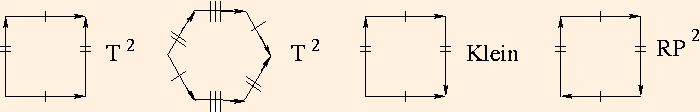The two tori represent rectangular and hexagonal tilings of the Euclidean plane, respectively.

After identifying the single-hashed sides of the hexgonal torus, the resultant "penne" must be twisted 180 degrees before the other sides will match up. (Thurston, p. 5)
Omit the singly-hashed identification on the Klein bottle to make a Moebius strip. (Henle, p. 106, 111, 112)
If you want to construct these, use a large piece of cloth and safety pins. Make each identified edge pair a noticeably different length, and pin them in the order of longest to shortest. It helps to use an additional set of pins for the identified vertices to keep them organized as you pin.

A simple notation is often used in such constructions. Label each singly-hatched side "a", each doubly-hatched side "b" and each triply-hatched side "c" (etc.). Then, typically starting with an "a" side whose direction is clockwise, proceed clockwise; whenever the side's arrow is parallel to your clockwise direction, write the letter; when the side has an arrow which is in the opposite direction, write the letter as an inverse. Hence we have:

• rectangular torus: a b-1 a-1 b
• hexagonal torus: a b-1 c-1 a-1 b c
• Klein bottle: a b a-1 b
• RP2: a b a b
Since the boundary of a Moebius strip is S1, we can remove a B2 from an S2 and glue in a Moebius strip to obtain RP2. Do it twice to obtain a Klein bottle. This operation is termed adding a cross-cap.
A sphere with g cross-caps has χ = 2 - g. (Edelbrunner and Harer, p. 34, 36)
• The torus can tile the Euclidean plane because at each vertex, four tori meet, and the interior angles add up to 2π. Similarly with the hexagonal torus: at each vertex, three tori meet, and again, the sum of the interior angles is 2π. For surfaces of higher genus (often called handlebodies), a Euclidean tiling is not possible. But a tiling of H2 is.

Consider a surface of genus 2. A two-holed torus can be constructed using identifications on an octagon:

a b a-1 b-1 c d c-1 d-1
(Another construction identifies parallel sides; this can also be done with a decagon to produce a genus-2 torus.)

An interior angle of a regular octagon is 3π/4. At each vertex, two octagons is not enough and three is too many. But as we see below, χ for the genus-2 torus is -2, which means that it can only be given a hyperbolic structure. When the octagon is embedded in H2, the sides become curved; by an appropriate choice of size for the octagon, the interior angles will be π/4, and the octagon can then be used to tile H2.

(Thurston, section 1.2)

• An identification of the sides of a 4g-gon of the form
a1 b1 a1-1 b1-1 ... ag bg ag-1 bg-1
will produce a genus-g torus. There are many identifications which will produce the same handlebody. In most cases twists (as in the hexagonal torus) will be required

An identification of the sides of a 2g-gon of the form

a1 a1 ... ag ag
will produce a non-orientable genus-g surface. All non-orientable surfaces can be obtained this way. Any two non-orientable closed surfaces with the same χ are homeomorphic.

(Thurston, p. 26)

• surfaceb1b2χπ1
E20120
H20120
annulus110Z
cylinder110Z
disc0110
S2012 (4)0
T2210 (2)ZZ
Riemann surface genus g2 g12 - 2 g (4) Z 2g | aba-1b-1cdc-1d-1... = 1 (Bott and Tu, p. 240)
Moebius strip100Z
Klein bottle2 (3)1 (3)0 (2)ZZ | abab-1 = 1
RP2001 (2)Z2
connected sum of n RP2n - 102 - n (4)Zn | aabbcc... = 1 (1)
non-orientable surface genus gg - 1 (5)0 (5)2 - g
(Gray, p. 46 (1)) (Henle, p. 167 (2), 193 (3), 295 (4)) (Hu, p. 28 (5))
• If P is an irreducible (unfactorable) polynomial of two complex variables, P=0 describes a Riemann surface. xn + yn = 1 for n>1 describes a Riemann surface of genus ½(n-1)(n-2).

The Uniformization Theorem states that a simply connected Riemann surface can be conformally mapped into exactly one of S2 (if χ > 0), C (if χ = 0) or B2 (if χ < 0).

The group of conformal automorphisms of a Riemann surface is discrete IFF π1 is nonabelian. For g ≥ 2, it has at most 84(g-1) elements.

Every Riemann surface whose π1 is nonabelian admits a hyperbolic metric compatible with its complex structure. It has finite hyperbolic area IFF it is a closed surface with n punctures (open discs removed) and 2g+n≥3.

A Riemann surface admits a positive-definite metric of the form ds2 = E du2 + 2 F du dv + G dv2. Such a metric has area element √ (E G - F2) du ∧ dv.

(Marden, sections 2.7, 2.9)

#### Classification

• Every oriented, closed 2-manifold is the quotient of either S2, E2 or H2, by a discrete subgroup of isometries acting freely on it. (Borisenko, p. 2)

Every closed 2-manifold is homeomorphic to S2, a connected sum of tori, a connected sum of tori and one projective plane, or a connected sum of tori and one Klein bottle. (Averett, p. 4)

Every compact, connected 2-manifold is topologically equivalent to a sphere (2-cell notation aa-1), a connected sum of tori (aba-1b-1cdc-1d-1...), or a connected sum of projective planes (aabbccdd...). Every compact, connected 2-manifold with boundary is equivalent as just stated, with some finite number of discs removed. (Henle, p. 122, 129) (Christenson, p. 439)

Every compact connected surface is diffeomorphic to S2 - some number of B2, with either I2 or Moebius bands connecting the holes.

Two surfaces are diffeomorphic iff they have the same genus, Euler characteristic and number of boundary components.

Two surfaces are diffeomorphic iff they have the same Euler characteristic and number of boundary components, and are both either orientable or non-orientable.

Every connected compact orientable 2-manifold is diffeomorphic to the surface obtained from an orientable surface of genus 1 - (χ + δ) / 2 by the removal of δ disjoint B2. (Hirsch, p. 189, 205, 207)

Every open surface admits a hyperbolic structure. (Scott, p. 421)

• The Euler characteristic of a surface can be computed from its triangulation as
χ = #vertices - #edges + #faces
A closed surface has hyperbolic, Euclidean or spherical (elliptical) structure iff its Euler characteristic is negative, zero or positive, respectively.
This holds for surfaces in general, except that surfaces homeomorphic to R2, S1R or the Moebius strip can be given both Euclidean and hyperbolic structures. (Thurston, p. 28, 118)
• Removal of an open disc reduces the Euler characteristic by 1.

The Euler characteristic of a surface formed by gluing two surfaces with boundary along a boundary component is the sum of the original Euler characteristics.

Note that RP2 is equal to a Moebius strip glued to a disc.
The Euler characteristic of the union of two surfaces is equal to the sum of their Euler characteristics minus the Euler characteristic of their intersection.

A sphere with g handles, c cross-caps and d open discs removed has Euler characteristic

χ = 2 - 2g - c - d

#### Isometries

• Moebius transformations on E2 can be written as
f(z) = (a z +b) / (c z + d),
where a, b, c and d are complex numbers subject to a d ≠ b c (the usual normalization is a d - b c = 1, which makes the group isomorphic to PSL(2,C)). These are the most general functions on E2 which map circles to circles.
f(z) is often represented as a matrix:
 a b c d
(also denoted ((a,b),(c,d))). If the trace of f(z) (Tr(f) = a+d) is

• <-2 or >2, f is loxodromic: a screw motion (conjugate to z → λ2z, |λ|>1);
• <-2 or >2 and real, f is hyperbolic: a loxodromic transformation with λ>1 (this term sometimes is used to include loxodromic);
• >-2 and <2 and real, f is elliptic: a rotation (conjugate to z → e2iθz, θ ≠ π; NB: z → -z is conjugate to z → 1/z);
• equal to ±2, f is parabolic: a Euclidean translation on the sphere at ∞ (conjugate to z → z+1).
A parabolic map has a single fixed point; a loxodromic, hyperbolic or elliptic map has two fixed points.
Some convenient trace identities are:

• Tr(f) = Tr(f-1)
• Tr(gfg-1) = Tr(f) (Tr is unchanged by conjugation)
• Tr(product) is unchanged under cyclic permutation of the product
• Tr(fg) = Tr(f-1g-1)
• Tr(fg) + Tr(f-1g) = Tr(f) Tr(g)

If Tr(f) = 0, f2 = I (so f is elliptic).

Conjugation can be used to effect a coordinate transformation on an iterated Moebius map {z, f(z), f2(z),...}.
The set of all points in E2 that can be reached by iteration of the maps in a Moebius sub-group G is called the regular set (Ω(G)) of the group. It has either one, two, or infinitely many connected components, each of which is either simply connected or contractible (to a point).

Λ(G) ≡ E2 - Ω(G) is called the limit set. These are the fixed points of G. If G has only elliptic elements, it is finite and Λ(G) is empty. If Λ(G) is not empty, it has one, two, or uncountably infinite points.

In the latter case it is a closed set, whose components are either topologically circles, fractal sets, or totally disconnected (every component is a point). (Marden, sections 2.4.1, 2.8)
(Series, p. 8)
Considering the quotient space obtained by Ω(G) / G.
It turns out that if G has no elliptic elements, the quotient space is the boundary of a hyperbolic 3-manifold M = H3 / G, where π1(M) = G and ∂M ≡ Ω(G) / G. If G contains elliptic elements, M is an orbifold. Unless specified below, we will assume G contains no elliptic elements.

The fundamental group of ∂M is isomorphic to G, which means the Kleinian groups constitute representations of the fundamental group for a given quotient space topology.

Restricting ourselves to 2-generator groups, generators a and b with inverses A and B, respectively:

• if G is a translation, ∂M is T2;
• if G is a hyperbolic group of rank n operating on 2n disjoint Jordan (simple plane closed) curves (with disjoint interiors) such that each group element maps the inside of one curve to the outside of a second, and the inside of the second to the outside of the first (such curves are paired), ∂M is a surface of genus n (G is a Schottky group), and Λ(G) is totally disconnected: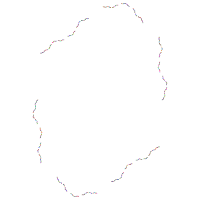• if G is a hyperbolic group of rank two operating on mutually tangent paired curves (a paired to A, b paired to B, as below), whose points of tangency are fixed, and whose commutator abAB has trace -2, ∂M is a surface of genus two with a cusp pinch (puncture); a pair of once-punctured tori: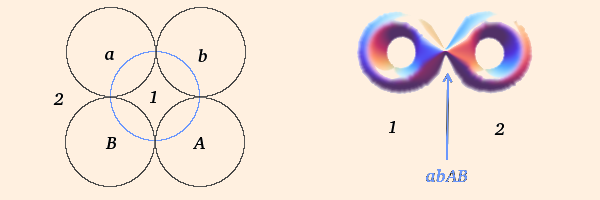The limit set passes through the points of tangency, which are fixed by cyclic permutations of abAB, and the group is called a quasifuchsian group. If the curve is a circle (as above) the group is Fuchsian and the traces of all the group elements are real.

The region exterior to the paired curves is made up of two disjoint tiles (labeled "1" and "2" above), which become the two sides of the torus separated by the puncture. All quasifuchsian groups yield the same type of quotient surface.

rank 2 with 1 parabolic → genus 2 with 1 element of π1 pinched → 2 once-punctured tori

Λ(G) of such a group (with Tr(abAB) = -2) is a closed curve: a quasicircle, which separates Ω(G) into two disjoint regions; one is tiled by the ideal quadralateral "1", the other is tiled by the ideal quadralateral "2":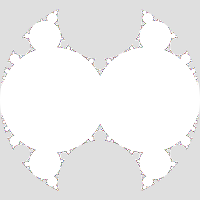The images here and below are the results of randomly iterating nontrivial products of a, b, A and B, up to 200 factors each, on the fixed points of a, b, A, B, abAB, AbaB, BabA and ABab, for a reasonably large number of iterations on each fixed point.
In general, if tr(abAB) = 2, a and b share a fixed point; a point fixed by a parabolic operator induces a puncture on ∂M, and each puncture increases the dimension of π1 by 1;
• if G is a parabolic group of rank two (a and b are both parabolic) operating on two disjoint pairs of tangent circles (one pair for each generator), ∂M is a surface of genus two with two punctures, equivalent to a sphere with four punctures: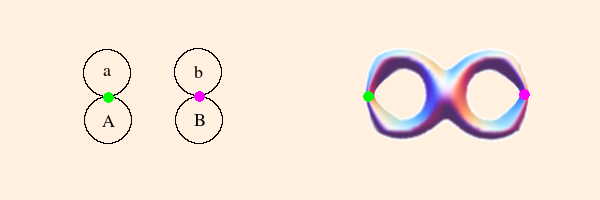The green point is the fixed point of a, and the magenta point is the fixed point of b. The region external to the circles corresponds to the surface of the 4-punctured sphere.

rank 2 with 2 parabolic → genus 2 with 2 elements of π1 pinched → 4-punctured sphere

Using the Riley parameter λ, we have a = ((1,2),(0,1)) and b = ((1,0),(λ,1)). Tr(ab) is 2(1+λ) and Tr(abAB) is 2(1+2λ2). The Riley slice is the corresponding 1-complex-dimensional slice of Teichmuller space for groups of two generators. The only point of contact with the Maskit slice (below) is the point λ=i, corresponding to the (0,1) gasket (μ=-2i).

The Riley slice is symmetric about both the real and imaginary axes. Here is a portion of the limit set for λ=0.05+0.93i: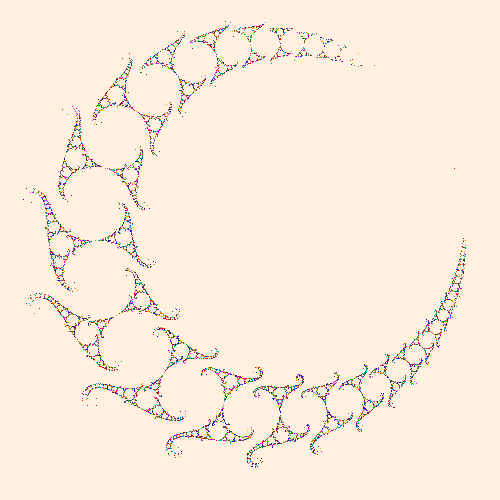To make this image, a and b were conjugated by ((i,-1),(1,-i))/√2 so that the points at real ∞ have been translated to (0,i) and the origin has been translated to (0,-i).

• if G is a parabolic group of rank two (a and b are both parabolic) operating on mutually tangent paired curves (as below) with Tr(abAB) = -2, ∂M is a surface of genus two with three punctures, equivalent to a pair of triply-punctured spheres: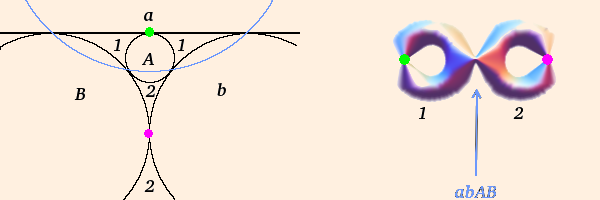As drawn, the radius of circle "a" is infinite; the green point is the fixed point of a, and the magenta point is the fixed point of b.

rank 2 with 3 parabolic → genus 2 with 3 elements of π1 pinched → 2 thrice-punctured spheres

For each additional independent parabolic element (besides abAB), one half of the regular set closes up into a set of tangent discs: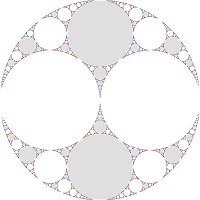The sets of tiles (on each side) are disjoint from each other.
When constructing the quotient space ∂M, all of the gray discs are identified, and the white discs will similarly be identified
(the gray region outside the limit set is a disc after adding in the point at ∞).
NB: The map maps circles, not discs; here we have colored them in to facilitate identification.
The circles are also iteratively mapped, so not all circles which should be gray are necessarily colored in.

We will call such groups gasket groups (also called double-cusp groups).

An acylindrical manifold is boundary incompressible and contains no essential cylinders. Such a manifold M of genus g with n punctures has 3g+n-3 simple loops which divide M into 2g+n-2 "pairs of pants", and whose corresponding group elements can become parabolic in the algebraic limit. Hence each of the loops can be pinched to become a puncture, and each pair of pants becomes a component of ∂M.

(Marden, section 5.3)

• if G is a modular group of rank two (all matrix elements ∈ Z), with both generators and their product parabolic, operating on paired curves (as below), ∂M is a joined pair of triply-punctured spheres: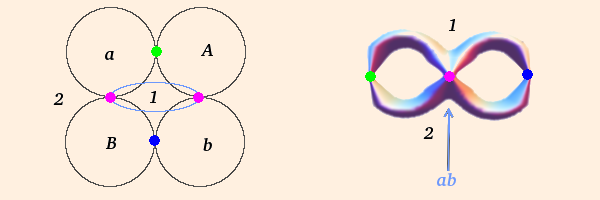(Here, the blue circle represents the actions of ab and ba.)

This modular group is unique up to conjugation, and the space is rigid (Marden, section 2.10);

• if G is a group of rank two where there exist group elements which are parabolic permutations of apBq (p and q relatively prime integers), ∂M includes a once-punctured torus component with a (p,q) Dehn twist.
Here, only one side of Ω(G) has closed up into a set of tangent discs; the part of ∂M corresponding to them is a triply-punctured sphere. The other side is simply connected, and its portion of ∂M is the once-punctured torus: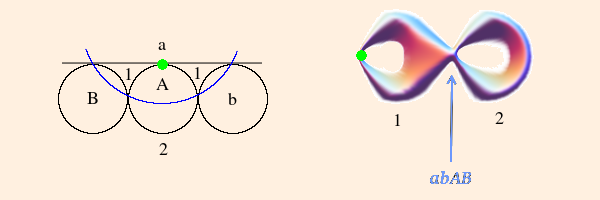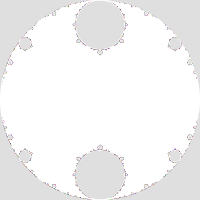rank 2 with 2 parabolic → genus 2 with 2 elements of π1 pinched → 1 once-punctured torus plus 1 thrice-punctured sphere
G is a single-cusp group.

An apBq group element can be found by constructing the set starting with {1}, adding q or subtracting p so that the elements are always positive and never greater than p+q, until you return to 1. The group element is the product of a's and B's created from writing an a for each subtraction and a B for each addition (in reverse order). For instance, the 2,5 set is

{1, 6, 4, 2, 7, 5, 3, 1}
and the corresponding group word is aaaBaaB. This is simply an arithmetic prescription for a consistent winding of q a's and p B's around the torus.

If we want G to be a gasket group, we will force Tr(b) to be 2 and look for a p,q element to be the other parabolic element (abAB will be parabolic with our parameterization). ∂M will then be a pair of triply-punctured spheres.

Parameterizing a by the Maskit parameter μ we have a = ((-iμ, -i), (-i, 0)) and b = ((1, 2), (0, 1)). The trace of the 2,5 group element is

-i5 - 4μ4 + 9μ3 - 12μ2 + 9μ - 4),
which must be ±2, from which we can obtain μ.

• Since -1 < im(μ) < 1 cannot yield a discrete group,
• and μ and its complex conjugate yield conjugate groups,
• we need only consider μ with im(μ) ≥ 1
• (im(μ) > 2 always yields a discrete group).

We therefore have three possible solutions: 0.375189 + 1.30024i, 1.06548 + 1.2824i and 0.766588 + 1.64214i. When generating Λ(G)s of the first two, one obtains chaotic, overlapping patterns characteristic of non-discrete groups; but the last one yields a discrete group (see below).

For p or q < 0, begin with -p,1, for which the parabolic word is abp, and 0,1 (parabolic word a). For any p,q find relatively prime integers r,s for which ps - rq = ±1. A new parabolic word will be

w(p+q),(r+s) = wp,qwr,s.
So, for example, w-1,2 = w-1,1w0,1 = aba.
(This construction works for positive p and q as well.)
p,q and -p,-q will produce the same word.
It is instructive to look at the discrete groups associated with the first few values of p and q. There are only 9 independent ones for p or q ≤ 5 because translations of μ by ±2k (k ∈ Z) are equivalent; also, the negative of the complex conjugate of μ yields the same limit set, but reflected in the imaginary axis. For each limit set below, the associated parabolic word is in parentheses:

 0,1 (a)0,3 (aaa)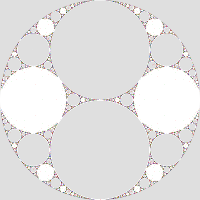0,4 (aaaa)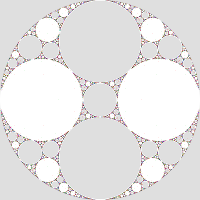0,5 (aaaaa)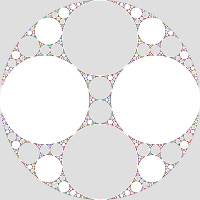1,2 (aaB)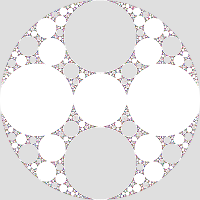1,3 (aaaB)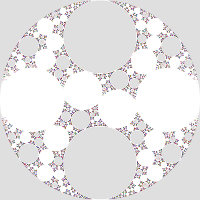1,4 (aaaaB)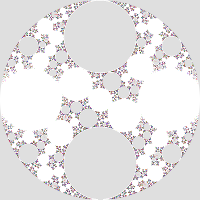1,5 (aaaaaB)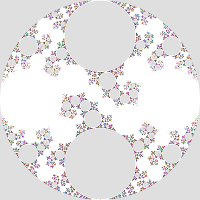2,5 (aaaBaaB)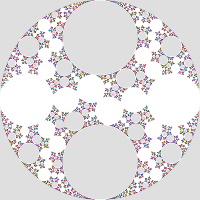±1,1 and ±2,1 and ±3,1 and ±4,1 and ±5,1 are translations of 0,1 (0,2 is the same as 0,1);
-5,2 and -3,2 and -1,2 and 1,2 and 3,2 and 5,2 are equivalent under translation;
-5,3 and -2,3 and 1,3 and 4,3 are equivalent under translation;
-4,3 and -1,3 and 2,3 and 5,3 are equivalent to 1,3 under reflection (and translation);
-3,4 and 1,4 and 5,4 are equivalent under translation;
-5,4 and -1,4 and 3,4 are equivalent to 1,4 under reflection (and translation);
-4,5 and 1,5 are equivalent under translation;
-1,5 and 4,5 are equivalent to 1,5 under reflection (and translation);
-3,5 and 2,5 are equivalent under translation; and
-2,5 and 3,5 are equivalent to 2,5 under reflection (and translation).
So we can generalize that ±p,q are equivalent under reflection, and that p+kq,q (k ∈ Z) are equivalent under translations.
Note that 0,3 and 0,4 and 0,5 are non-free; in each case the associated parabolic word is -I (where I is the identity matrix). The limit sets do not bound discs; they are fractal dust. The same holds true (up to sign) for all 0,q for q > 2.
• Limit sets for groups which are not discrete are essentially random; given an infinite amount of time, the program will eventually fill the complex plane: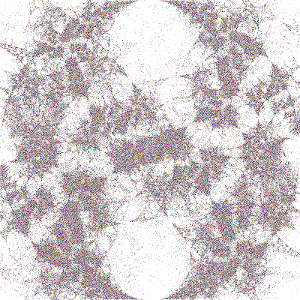• There are relatively prime integers r and s such that ps - qr = ±1. If α is a simple geodesic loop with slope p/q and β is a simple loop with slope r/s, the geometric intersection number
ι = | ps = qr |
is the number of times α crosses β on the quotient surface. (Marden, problem 2.6)
• Values of μ associated with irrational windings produce singly (below left) or doubly (below right) degenerate groups: one or both sides of Ω(G) has zero area, and Λ(G) (of dimension 2) completely fills the region(s):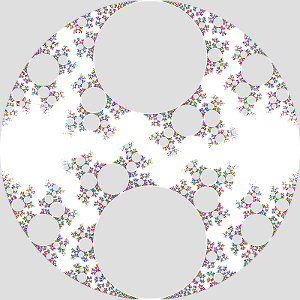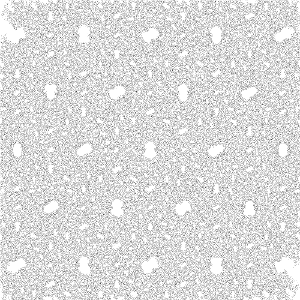In both cases, Λ(G) is incomplete; on the left, given infinite time the program would completely fill in the white region outside the gray circles (which constitute, with the exterior region, the non-degenerate part of Ω(G)); on the right, given infinite time the program would fill the entire complex plane.

Degenerate groups are discrete and geometrically infinite (but M(G) may have finite volume).

The limit set of a doubly degenerate discrete group is the entire sphere at ∞. This is also true for closed hyperbolic manifolds. (Thurston, p. 172)
The irrational slope of the winding is the ending lamination for the associated cusp. (Marden, problem 2.6)
• Groups for which some product of generators is equal to the identity are not free; they are not quasifuchsian (their limit sets are fractal dust).
• Groups with three or more generators and tangent paired curves yield more complex quotient spaces (tori with multiple punctures, spheres with more punctures, etc.)

For example, let

a = ((1 + 2i, 4), (1, 1 - 2i)),
b = ((1 + 1.5i, 1), (2.25, 1 - 1.5i)) and
c = ((-13 - 4i, 8i), (-12 + 16i, 11 + 4i)).
a and b have trace 2, and c, aB and cbCB have trace -2. This group corresponds to Mumford et. al., figure 11.6; the limit set is conjugate to their figure 11.7: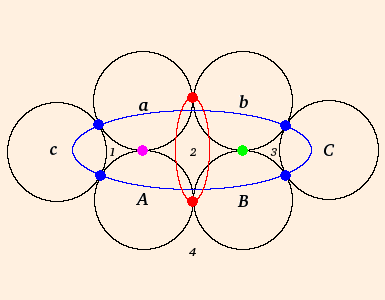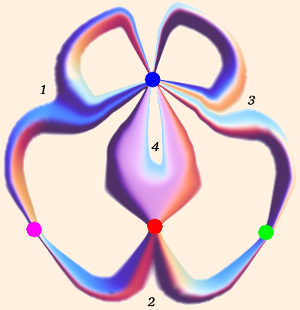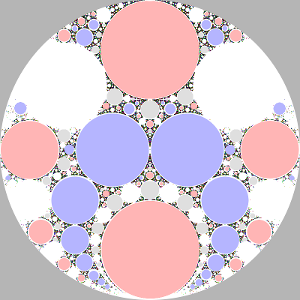The magenta and green points correspond to the fixed points of a and b, respectively; the red circle corresponds to the action of aB, and the blue circle corresponds to the action of acBC. The quotient surface is four triply-punctured spheres. The limit set has four mutually disjoint sets of components, corresponding to the four numbered tiles.

rank 3 with 6 parabolic → genus 3 with 6 elements of π1 pinched → 4 thrice-punctured spheres
Groups exist for which any of the disjoint sets of circular components can become degenerate (these are called partially degenerate groups).
(Mumford et. al., chs. 3, 4, 6, 7, 9, 10)

For any two sets of three points, there is exactly one element of PSL(2,C) which maps the first set to the second. (Thurston, p. 87)

• The moduli space of a surface is the quotient of the space of metrics admissible to the surface, by the action of the diffeomorphisms of the surface (Diff).
The Teichmuller space restricts the diffeomorphisms to those homotopic to the identity by a homotopy which takes the boundary into itself at all times (Diff0).
The mapping class group is Diff S / Diff0 S. Therefore the moduli space is the quotient of the Teichmuller space by the mapping class group.

If a surface has empty boundary, two structures of S are equivalent in moduli space iff they have the same holonomy group (up to conjugacy). They are equivalent in Teichmuller space iff they have the same holonomy map.

The mapping class group of T2 is PSL(2,Z) (the projective special linear group acting on pairs of integers).

The Teichmuller space of a compact surface that admits a hyperbolic structure is homeomorphic to R 3 |χ|.

The maximum number of disjoint, non-parallel simple closed curves on a hyperbolic surface is 3g - 3. Cutting the surface along the corresponding geodesics divides the surface into 2g - 2 surfaces homeomorphic to S2 - 3 B2 (pairs of pants). The Teichmuller space of the original surface corresponds to the degrees of freedom defining the lengths of the boundary components of the pants, along with the number of twists with which the pants are glued back together.

If there are n punctures, the dimension is 3 |χ| - n (or 6g + 2n - 6). (Marden, sect. 2.10)

For groups of two generators, the quotient space has χ = -2, so the Teichmuller space is 6-dimensional. We can parameterize it by the complex traces Tr(a), Tr(b) and Tr(ab).

The Maskit slice is a 1-complex-dimensional slice of Teichmuller space corresponding to Tr(b) = 2 and Tr(abAB) = -2. The slice has translational symmetry because Tr(ab) = Tr(a) ± 2k, k ∈ Z. From our considerations above, we need only examine the region 0 ≤ Re(μ) < 2, 1 ≤ Im(μ).

On the left, Im(μ) = 2 and Re(μ) varies from 0 to 1.9; on the right, Re(μ) = 1 and Im(μ) varies from 4 to 1.5: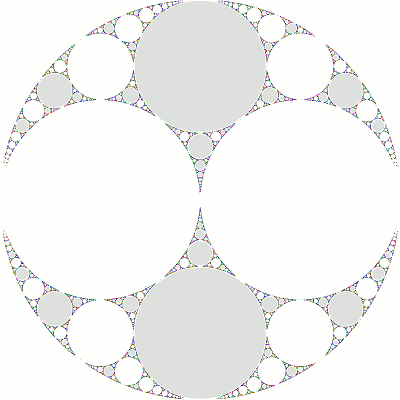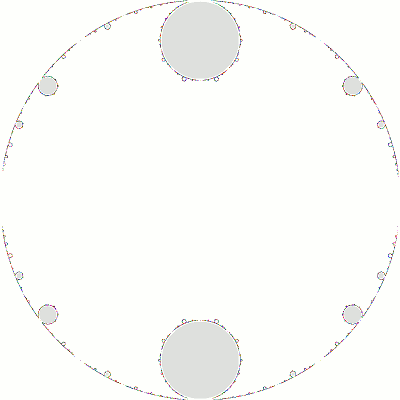The region outside the unit circle in these plots should be gray, but we have left it white to better show how the limit set "bleeds" out of the unit circle.
As Im(μ) decreases (right), the first seven frames correspond to single-cusp groups: their topology is a triply-punctured sphere (gray discs) plus a (simply-connected) once-punctured torus (white region). As the torus "closes up" (the last remaining loop of π1 becomes "tighter"), the group approaches a double-cusp group. The eighth frame is the (1,2) gasket, and the last three frames are non-discrete. The latter show an increasing "overlap" in the limit sets, but with sufficient time, the limit set will fill all three frames.

The "essential" portion of the slice is shown here (with some groups identified by (p,q):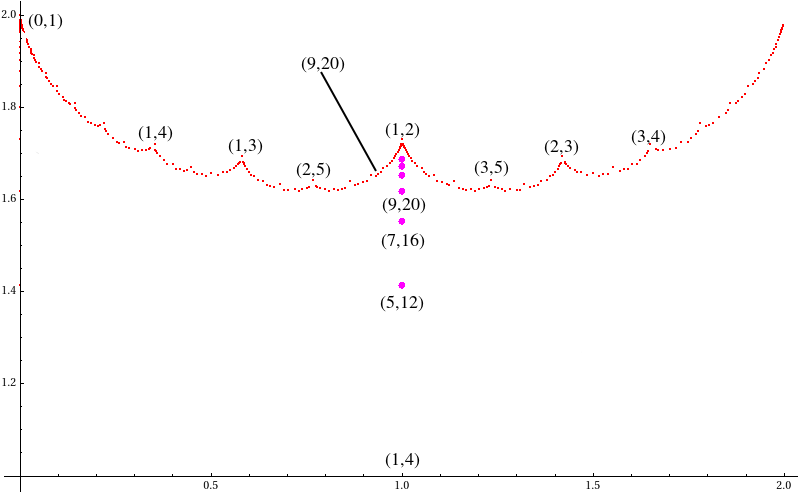The red points indicate the gasket groups on the Maskit boundary. Groups with values of μ above the boundary are single-cusp groups. Groups below the boundary are almost all non-discrete, with one class of exceptions: the magenta points are non-free groups. Note that a single value of (p,q) (i.e., 9,20) can have solutions to its trace equation which are a gasket and a non-free group (9,20 also has eight non-discrete groups). The non-free groups plotted above are (5,12), (7,16), (9,20), (11,24), (13,28) and (15,32).

Note that the region 0 ≤ Re(μ) < 2 appears to be symmetric about the line Re(μ) = 1. This is due to a combination of symmetries g(μ) ≈ g(μ*) ≈ g(-μ*) ≈ g(μ + 2), or equivalently, g(μ) ≈ g(2 - μ), where here "≈" denotes equivalence up to conjugacy (the "mirror image" groups labeled above are all conjugate).

The slice also shows a pattern in the ratio p/q: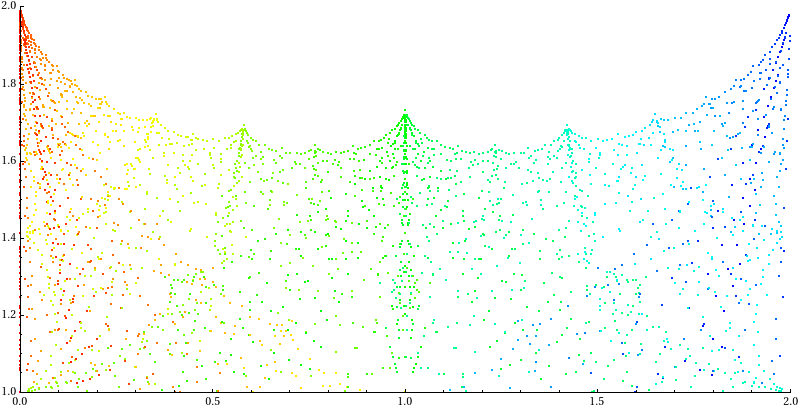Here red corresponds to p/q = 0 and blue corresponds to p/q = 21/22 (the highest ratio computed for this data set).

(Mumford et. al., ch. 10)

The mapping class group of a closed surface is isomorphic to the outer automorphism group of its fundamental group.
The outer automorphism group is the conjugacy class under diffeomorphisms of maps of π1 into itself.
(Thurston, p. 260, 262, 264, 266, 271, 276, Thurston Notes, pp. 89-91)

## On 3-manifolds

• All compact orientable 3-manifolds are boundaries. (Greenberg, p. 240)
• Every 3-manifold can be obtained by gluing together the boundaries of two solid tori of some genus. This is called a Heegaard splitting. (Thurston Notes, pp. 1-3)

For a given 3-manifold M, the minimum genus for all possible splittings is the Heegaard genus. The rank of π1(M) cannot be larger than the Heegaard genus. (Marden, section 2.8.1)

• A 3-dimensional gluing of tetrahedra is a manifold iff
χ = #vertices - (2 #edges - 3 #faces + 4 #tetrahedra) / 2
is zero. So χ = 0 for any closed 3-manifold.
If X is a 3-space and it has k vertices vk,
χ(X) = k - ½ Σ χ(link(vk))
(Thurston, p. 122)
When identifying faces of polyhedra, the total number of faces must be even, and every identification must be orientation-reversing between pairs of faces. This produces an oriented manifold except near the vertices, which must be removed in order for the identification to produce a manifold. (Thurston Notes, p. 3)
• A closed 3-manifold is irreducible if every embedded S2 is the boundary of a B3.

If M is orientable and irreducible, then π2(M) = 0. (Scott, p. 483)

• A closed 3-manifold is prime if every separating embedded S2 is the boundary of a B3. The three main classes are
• S3 / Γ, where Γ is a finite subgroup of SO(4) acting freely by rotations; Γ = π1(M). If Γ is cyclic, M is a lens space.
A (p,q)-lens space is generated by Γ = ((e2πi/p,0),(0,e2πiq/p)). Two lens spaces with indices (p,q) and (p',q') are homeomorphic iff p=p' and either q=±q'(mod p) or qq' = ±1(mod p). (Ratcliffe, p. 342)
• S1 ⊗ S2, with infinite cyclic fundamental group. It is the only orientable 3-manifold that is prime but not irreducible, and the only prime orientable 3-manifold with nontrivial π2.
• aspherical manifolds, irreducible with infinite noncyclic π1.
Sphere / Prime Decomposition - every orientable closed 3-manifold has a finite connected sum decomposition into prime manifolds. (Borisenko, p. 8)
• Torus Decomposition - every closed orientable irreducible 3-manifold has a finite collection of disjoint incompressible tori (i.e., cusp tori) which decompose it into a finite collection of compact 3-manifolds with toral boundary, each of which is either torus-irreducible or Seifert fibered.
A Seifert fibration is a 3-manifold fibered by circles which are the orbits of a circle action which is free except on at most finitely many fibers.
(Borisenko, p. 6, 10)

#### Examples

• S2 ⊗ S1 is obtained by identifying corresponding points on a pair of S2, one inside the other; it can also be obtained by identifying corresponding points on two congruent solid tori. (Alexandroff, p. 11)
• S3 can be obtained by identifying the surfaces of two solid tori.
• The figure 8 knot complement is formed by the following identifications on a pair of ideal equilateral tetrahedra (whose vertices lie on the sphere at ∞), and removal of the vertex: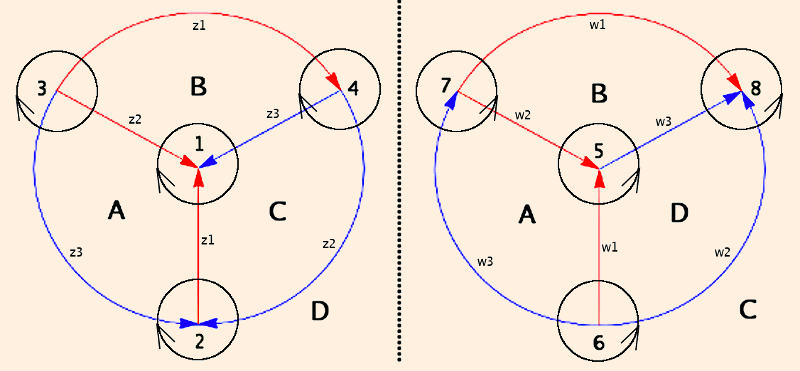The vertices of the original tetrahedra are numbered 1 through 4 and 5 through 8, all identified, and the faces (identified) are labeled A through D. All of the red edges are identified, as are all of the blue edges. The edge labels (zi and wi) are vertex invariants, each corresponding to the the opposite dihedral angle. The link of each vertex is denoted by the directed circles; since the tetrahdrea are ideal, the links are Euclidean triangles.
The vertex invariant of a vertex of a Euclidean triangle in the complex plane is the ratio of the adjacent sides. Labeling the vertices clockwise,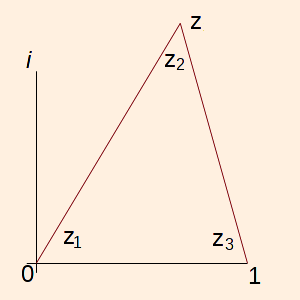• z1 = z / 1
• z2 = (z - 1) / z
• z3 = 1 / (1 - z)

Note that z1 z2 z3 = -1.

The identifications produce an object with 1 vertex, 2 edges, 4 sides and 2 tetrahedra; removal of the vertex (so that χ = 0) makes the object a 3-manifold.

By considering the neighborhood of the removed vertex, we see that the boundary is a torus (the grayed area is a fundamental domain for the torus, but the green area is the fundamental domain with respect to the knot):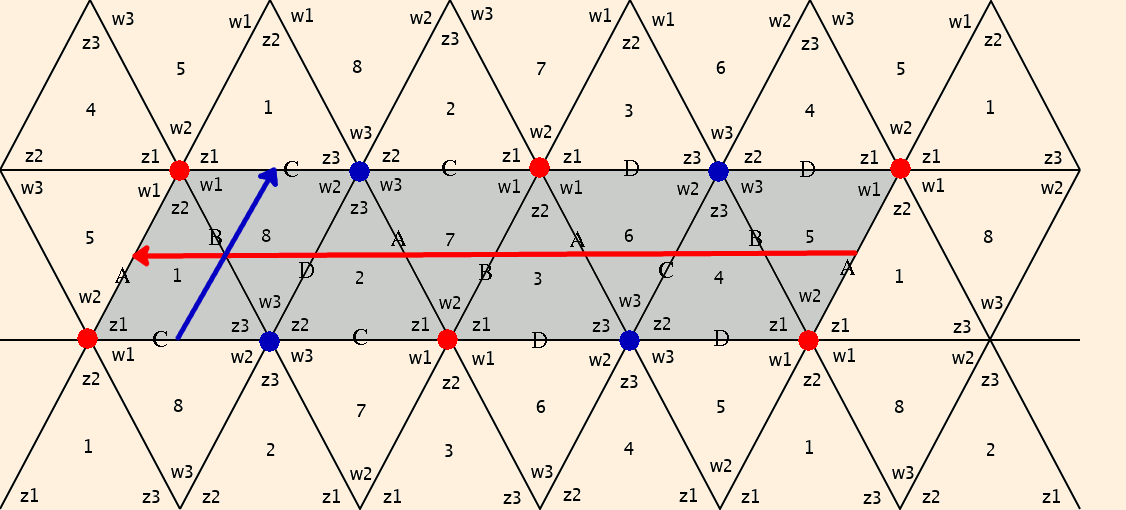Here, the numbers indicate the vertices, the vertex invariants are labeled, and each triangle represents the link of its associated vertex. The arrows correspond to the directed circles; rotation across a vertex invariant zi in a counterclockwise direction introduces a factor of zi in the numerator, while rotating across zi in a clockwise direction introduces a factor of zi in the denominator. The red line corresponds to the holonomy generator in the longitudinal direction of the torus (dH(l)/dl = z12/w12, after dividing by z12z22z32w12w22w32 ≡ 1), and the blue line correspond to the generator of holonomy in the meridianal direction ( dH(m)/dm = w1/z3).

This calculation used the "gray" domain. If we use the "green" domain, the meridian stays the same, but the longitude becomes
1/√z1 * w3 * z2 * w3 * 1/z2 * w2 * w3 * z2 * w3 * z3 * z1 * w2 * √z1 * -1
= - z1 z2 z3 w22 w34

= w32 / w12

= 1 / (w12 (1- w12))

(Neumann and Zagier, p. 329)
For the manifold to be complete, the products of the vertex invariants around any intersection of the link diagram must be 1:

• w12 w2 z12 z2 ≡ 1 and (equivalently)
• w2 w32 z2 z32 ≡ 1, or
• w (w - 1) z (z - 1) ≡ 1.
(These are often called gluing consistency conditions.)
In addition, completeness imposes two other conditions: dH(l)/dl and dH(m)/dm must both equal one (the holonomy must be trivial). The first condition tells us that z=w; the second determines z:
z (1 - z) ≡ 1
Since we require solutions such that Im(z) > 0 (Im(w) > 0) (all dihedral angles positive and ≤ π), z = (-1)1/3. Notes that this solution also satisfies the first completeness condition.
• The figure 8 knot complement is H3 / G, where G is generated by
a(z) = z / (z + 1),
(a = ((1,0),(1,1)) and is therefore parabolic.)
b(z) = z + ei π/3,
(b = ((1,ei π/3),(0,1)) and is also parabolic.)
and G is doubly degenerate. (Marden, section 3.14)

#### Geometrization Conjecture

• For the following, X is a connected and simply connected space, G is the maximal Lie Group of diffeomorphisms acting transitively on X, H0 is the compact identity component of the isotropy group of G, and there exists a compact manifold M and a discrete subgroup Γ of G such that
M = X / Γ. (Borisenko, p. 11, 16, 20, 22)
So X is the universal cover of M, and π1(M) = Γ.
Thurston conjectured that every oriented closed prime 3-manifold has a torus decomposition such that its components have one of the following geometric structures. It was proved by Perelman in 2003.
XS3H3E3H2RS2R SL(2,R)~NilSol
GSO(4)PSL(2,C)R3 ⊗ SO(3)OPS Isom H2 ⊗ Isom E1 OPS SO(3) ⊗ Isom E1SL(2,R)~ ⊗ R(1)(2)
H0SO(3)SO(3)SO(3)SO(2)SO(2)SO(2)SO(2){e}
ds212 + sin(θ1)222 + sin(θ1)2 sin(θ2)22 (dx2 + dy2 + dz2) / z2 dx2 + dy2 + dz2dx2 + cosh2x dy2 + dz2 2 + sin(θ)22 + dz2dx2 + dy2 - dz2 dx2 + dy2 + (dz - x dy)2 e2z dx2 + e-2z dy2 + dz2
isometry groupO(4)Moeb(E2)O(3) ⊗ R3Isom(H2) ⊗ RO(3) ⊗ R SL(2,R)~ ⊗ RNil ⊗ S1Sol
topologically =H2RR3R3
bundle structurenontrivial S1 over S2trivial S1 over T2trivial S1 over surface g > 1S1 over S2 nontrivial S1 over surface g > 1nontrivial S1 over T2nontrivial T2 over S1
Bianchi classIXV, VIIIIII(none)III, VIIIIIVI
topological invariantse ≠ 0e = 0 e = 0e = 0e ≠ 0 e ≠ 0
R6-60-220-1/2-2
Ra bRa b121202203/44

X"round" T3H3H3
ds2(ra - c2 rb + c2 c3 rc)2 dc12 + (rb - c3 rc)2 / (1 - c22) dc22 + rc2 / (1 - c32) dc32 dx2 + dy2 + dz2 - Σ xi xj dxi dxj / (1 + r2)dr2 + cosh2 r dx2 + sinh2 r dθ2
R2 c3 (ra - 2 c2 rb + 3 c2 c3 rc) / (rc (-rb + c3 rc) (ra - c2 rb + c2 c3 rc)), < 0-6-6
Ra bRa b(2 c32 (ra2 - 3 c2 ra rb + 3 c22 rb2 + 4 c2 c3 ra rc - 8 c22 c3 rb rc + 6 c22 c32 rc2)) / (rc2 (-rb + c3 rc)2 (ra - c2 rb + c2 c3 rc)2), > 0 1212

(bundle structure Anderson, p. 185)
(curvature invariants from Koehler)

• "~" denotes the Universal Cover.
• Nil = 3-dimensional Heisenberg Group (matrices of the form {{1,x,y},{0,1,z},{0,0,1}}).
In the above coordinates, geodesics beginning in the x-y plane lift into the z direction; similarly with the x-z and y-z planes. In the x and z directions, straight lines are geodesics, but not in the y direction.
• Sol = Lie Group with topology RR2 such that (t,(x,y))→(etx,e-ty); isotropy group is trivial.
In the above coordinates, geodesics in the x-z or y-z planes stay in those planes; geodesics in the x-y plane do not. Straight lines in the z direction are geodesics, but not in the x or y directions.
• "OPS" denotes Orientation Preserving Subgroup.
• The OPS of Moeb(E2) is PSL(2,C), or Projective SL(2,C) (scalar multiple are identified).
• (1) semidirect product of X with S1, acting by rotations on the quotient of X by its center.
The center of a group is the set of all members which commute with every member of the group.
• (2) extension of X by an automorphism group of order 8.
• e denotes the Euler number of the associated Seifert bundle.
• For T3, the ri parametrize the radii of curvature (with ra > rb + rc and rb > rc) and the ci the cosines of the angles for each of the S1 factors.
Incompressible tori (g = 1 only) are obstructions to the existence of a geometric structure, and there are no geometries which interpolate continuously between any of the eight structures. So the idea is to control the metric degeneracy in the decomposition regions by using the Ricci Flow:
d g(t) / dt = -2 Ric(g(t)) + λ(t) g(t)
to approach the singularities and then excise them. To get a feel for how it works, consider Einstein metrics, which are "fixed points" of the flow. For metrics of positive curvature, the flow contracts the metric (to a point in finite time, unless the flow is volume-normalized); for metrics of negative curvature, the flow expands the metric forever. The Ricci Flow preserves isometries since it is diffeomorphism invariant, so the geometric structure is valid arbitrarily close to each singular region. Perelman showed that for an arbitrary 3-manifold, only a finite number of surgeries are required to decompose the manifold into sub-manifolds, each of which has one of the eight geometric structures above. (Anderson, p. 185-9, 191-2)

S3, E3, SL(2,R)~, Nil and Sol are unimodular Lie groups. (Scott, p. 464, 468, 470, 478)

#### Classification

• Any metric of curvature +1 on a spherical 3-manifold is unique up to isometry. (Borisenko, p. 13)
• If M is a smooth manifold, the mapping torus of a diffeomorphism φ: M → M is the manifold obtained from M ⊗ I by identifying the ends of the cylinder via φ.

Every closed Euclidean 3-manifold is the quotient of T2R by the action of a discrete group Γ:

• T3, the mapping torus of T2 by a translation
• the mapping torus of T2 by the cyclic group of order 2 (C2)
• the mapping torus of T2 by C3, C4 or C6, each of which have two forms distinguished by handedness
• Γ = C2 * C2, acting as rotations around perpendicular axes
The remaining manifolds are not orientable:
• Γ = C2 * C2, acting as glide-reflections in a plane, of index 2 or 4
• Γ = C2 * C2, acting as one screw motion and one reflection, in orthogonal planes, with two possible spacings
Three of these are Klein bottle bundles over circles. (Weeks (Shape), p. 248)

(Thurston, pp. 159, 233-238)

All 10 closed Euclidean 3-manifolds are finitely covered by the 3-torus. (Scott, p. 448)

• Every elliptic 3-manifold is orientable.

Every elliptic 3-manifold is either a lens space, or the quotient of RP3 by one of the following groups:

• the product of the dihedral group (D2n of a regular n-gon), the tetrahedral group (order 12), the octahedral group (order 24) or the icosahedral group (order 60), with a cyclic group of order relatively prime to the order of the first factor
• a subgroup of index 3 in the product of the tetrahedral group and the cyclic group C3m, m odd
• a subgroup of index 2 in the product C2m ⊗ D2n, n even and m and n relatively prime (D is the dihedral group)

(Thurston, pp. 243, 250)

• There are seven manifolds with S2R structures: S2R and two line bundles over P2 (noncompact); two S2 bundles over S1, P2 ⊗ S1 and P3 # P3 (compact). The latter is the only closed 3-manifold with a geometric structure that is a non-trivial connected sum. (Scott, p. 457)
• M has a Sol structure iff M is either a bundle over S1 with fiber the torus or Klein bottle, or is the union of two twisted I-bundles over the torus or Klein bottle. (Scott, p. 477)
• A closed 3-manifold possesses a geometric structure other than H3 or Sol iff it is a Seifert fiber space.

3-manifolds with geometries E3, H2R or S2R are, up to finite covers, trivial circle bundles over oriented surfaces of genus g, with g = 1, g > 1 or g = 0, respectively.

3-manifolds with geometries S3, SL(2,R)~ or Nil are, up to finite covers, nontrivial circle bundles over oriented surfaces of genus g, with g = 0, g > 1 or g = 1, respectively.

These are also twisted line bundles over S2, H2 and E2, respectively. (Weeks (Shape), p. 253, 254)
A closed 3-manifold possessing Sol geometry is finitely covered by a torus bundle over S1 with holonomy given by a hyperbolic automorphism of T2 (an element of SL(2,Z) with distinct real eigenvalues). (Borisenko, p. 29)
• For H3/Γ,

• The minimum dimension of the Teichmuller space of a 3-manifold that admits a hyperbolic structure is -6 χ. (Thurston Notes, p. 88)
• if the manifold is compact, Γ is infinite, has no subgroup of finite index that is abelian, nilpotent or solvable, and does not have a normal, infinite cyclic subgroup;
• if the manifold is noncompact with finite volume, Γ does not have a normal, infinite cyclic subgroup.
• (Thurston, pp. 281, 283)

• Every orientable 3-manifold can be obtained from Dehn surgery, cutting a tubular neighborhood of a knot or a link and gluing it back together with a different identification.
A knot is a simple closed curve.
A link is a union of disjoint knots.
A knot complement has a cusp along each link component. (
Gukov, p. 17)
• Every compact 3-manifold contains a simple closed curve whose complement admits a noncompact hyperbolic structure of finite volume.

Every noncompact hyperbolic 3-manifold of finite volume can be decomposed into a finite number of ideal hyperbolic tetrahedra. (Callahan et al., p. 321)

The toral cross-section of a cusp can be seen as the open tubular neighborhood of the link components strung along the edges of an ideal tetrahedron. (Weeks)

• The measure of the dihedral angle of an ideal vertex is determined by the link (triangle → π/3, square → π/2, etc.). Suppose a manifold is constructed from a set of regular ideal polyhedra with their sides identified in pairs. If the original set had n edges, and the manifold has m, the manifold is complete if n/m times the dihedral angle = 2π. (Ratcliffe, p. 441)

The link of a cusp (topologically a torus or a Klein bottle) is triangulated by the links of the vertices with appropriate identification.

If the first homotopy group is generated by {xi} and the product of the vertex invariants along each x is Zi, the holonomy along a path aixi is Ziai (implicit summation). If the manifold is complete, the holonomy is trivial.

(Ratcliffe, section 10.5)

• The Dehn filling of a 3-manifold M with torus boundary is the gluing of a solid torus to the boundary of M. Dehn fillings are parametrized by H1(∂M, Z) (without regard to orientation). The class parametrizing the filling is called the slope. (Dunfield, p. 419)

(p,q)-Dehn surgery is a Dehn filling where the meridian wraps p times around the meridian and q times around the longitude (p and q relatively prime, q ≠ 0).

The meridian is the homotopy generator of "shorter" radius (if the torus is filled, it bounds an embedded disc). The longitude is the homotopy generator of "larger" radius (orthogonal to the meridian, intersecting it once).
(p,q) and (-p,-q) surgeries produce the same manifold.
(p,q) Dehn surgery on a hyperbolic manifold will produce another hyperbolic manifold except in finitely many (at most 12) cases. (Marden, section 4.10)
The result of Dehn surgery always has a cyclic fundamental group. (Thurston Notes, p. 2)

Every 3-manifold can be obtained from Dehn filling of a finite-degree covering space of the Borromean Rings: they are a universal link.

The Borromean Rings are 3 linked S1 such that cutting any one unlinks the other two: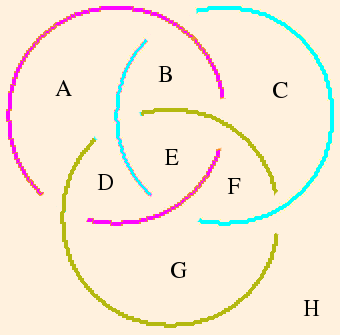and the 946 knot:are also universal.

(Thurston Notes, p. 57, 61) (Thurston, p. 131) (Thurston How..., p. 2569) (Hilden)
• The homology groups of any two knot complements with (p,q) and (p',q') Dehn fillings are the same if p = p'. (Meyerhoff, p. 47)

π1(knot complement) = Z iff the knot is trivial. (Thurston Notes, p. 2)

• (p,q) is the Dehn surgery invariant of an incomplete manifold M.

For the figure 8 knot complement, the generator of translations in the meridianal direction is (from the link diagram above)

w1 / z3
= w1 (1 - z1)
and the generator of translations in the longitudinal direction is the product of the longitudinal holonomy generator with the square of the meridianal holonomy generator:
w22 w32 z14 z22 z32 * (w1 (1 - z1))2
= z12 (1 - z1)2.
(which by the gluing consistency conditions is equal to the result we obtained above.)
The gluing (completeness) conditions for (p,q) Dehn surgery can then be written as

• p ln|z (1-w)| + 2q ln|z (1-z)| = 0 and
• p arg(z (1-w)) + 2q arg(z(1-z)) = 2π.

where z = z1 is the vertex invariant of the first tetrahedron and w = w1 of the second.

(Ratcliffe, p. 497)

Consider the (1,2) Dehn twist of the figure 8 knot complement. We find z and w by requiring both that the original gluing condition be satisfied and that the product of one meridian traversal and two longitude traversals gives the identity:

• w (1-w) z (1-z) = 1
• w z4 (1-z)5 = 1

There are 16 solutions to these equations; only one satisfies the Dehn surgery gluing conditions and produces zi and wi in the interval (0,π):

• z = -0.243106 + 0.560237i and
• w = 1.35334 + 0.70357i.
 |z1| arg(z1) |z2| arg(z2) |z3| arg(z3) |w1| arg(w1) |w2| arg(w2) |w3| arg(w3) 0.61071 113.458 2.23268 42.2824 0.733397 24.2599 1.5253 27.4688 0.516168 35.8646 1.27014 116.667

(arg values in degrees)

Adding all of the interior angles in the torus boundary of the removed vertex, we obtain 1440 degrees. Therefore the torus is described by a 10-sided polygon: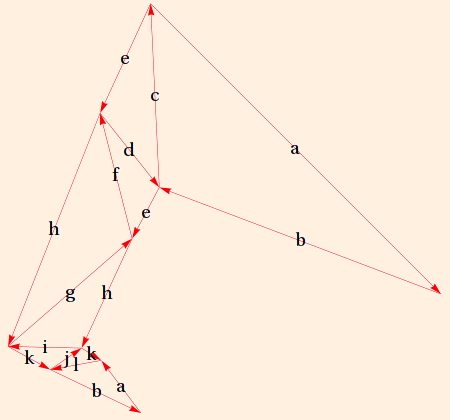Traversal of a meridian results in the length of a vector increasing by a factor of |w1 (1 - z1)| = 2.07978 and a rotation of arg(w1 (1 - z1)) = 3.20887 degrees counterclockwise; traversal of a longitude results in the length of a vector decreasing by a factor of |z12 (1 - z1)2| = 0.693412 and a rotation of arg(z12 (1 - z1)2) = 178.396 degrees: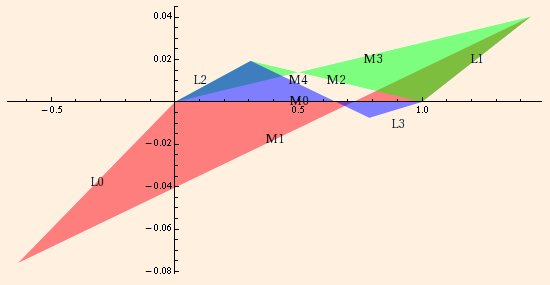Each "twisted quadrilateral" is a slice of the Dehn-filled cusp, with meridian and longitude edge labeling indicating traversal along two longitudes (red to green and green to blue) and one meridian (blue back to red). Since the manifold is now complete and compact, the red and blue quadrilaterals share a meridian edge (M0): traversal along one meridian and two longitudes brings you back to where you started. (See Weeks.)

• For a square-free integer d > 0:

1. PSL(2, Od) is a discrete subgroup of PSL(2, C). Let Γ be a torsion-free subgroup of finite index in PSL(2, Od). Then H3 / Γ is an oriented hyperbolic 3-manifold of finite volume.
2. Let D = d if d is 3 mod 4, otherwise D = 4 d.
3. Let (-D n) be defined by
• (-D n) = Πprime factors p (-D pi)
• (-D 1) = 1
• (-D 2) = 1 if -D is 1 mod 8, -1 if -D is 5 mod 8
• (-D p) = 0 if p is a factor of D
• (-D p) = 1 if -D is some square mod p, otherwise -1
4. The Lobachevsky function Π(θ) = - ∫0θ ln |2 sin u| du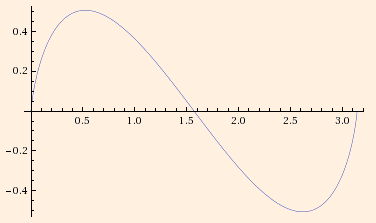5. The volume of H3 / Γ = (D / 12) Σk mod D (-D k) Π(π k / D) times the index of Γ in PSL(2, Od)

The volume of an ideal tetrahedron with dihedral angles α, β and γ is Π(α)+Π(β)+Π(γ). (Thurston Notes, pp. 160, 167-169)

• The volume of the figure 8 complement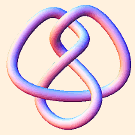is 6 Π(π/3) = 2.02988321282... (since an equilateral tetrahedron has dihedral angles of π/3).

The volume of the (1,2) Dehn-filled figure 8 complement is

Π(z1) + Π(z2) + Π(z3) + Π(w1) + Π(w2) + Π(w3)
= 1.3985088842...
• For the simple k-linked "closed" chain Ck (in which k S1s are linked, each to the next and the last to the first), let α = cos-1 (cos (π/k) / √2) and β = π - 2α.
Then the volume of the complement for k > 1 is
v(S3 - Ck) = 2 k (2 Π(β/2) + Π(α + π/k) + Π(α - π/k))
(Thurston Notes, p. 147, 167)
• The Weeks manifold, of volume 0.9427, appears to be the unique closed orientable hyperbolic 3-manifold of smallest volume. It is formed by a (2,1) Dehn fill on the "figure 8 sibling" (census manifold m003). (Gabai, Meyerhoff and Milley)
• Let M be a hyperbolic manifold formed by (p,q)-Dehn surgery on a knot complement. The volume of M is less than the volume of the original (cusped) complement, approaching it in the limit p2 + q2 → ∞.

Here are the volumes produced by (p,q) surgery on the figure 8 knot complement for p,q ≤ 20. Note that for (p,q) = (1,1), (2,1), (3,1) and (4,1), the resulting manifolds are not hyperbolic. The minimum volume (0.981369) is for the (5,1) surgery; the maximum volume shown (2.02326) is for (19,20) surgery; the limiting volume is 2.02988.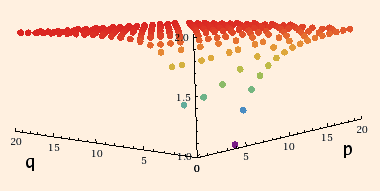(Gukov, p. 17) (plot data created by SnapPy)

• πk > 1 = 0 for any knot complement in S3. (Lickorish, p. 114)

#### Hyperbolic Manifolds

• A manifold M is atoroidal if every map of a torus which injects its fundamental group into M is homotopic to a map to a torus boundary component of M.

Every closed irreducible atoroidal 3-manifold with infinite fundamental group is hyperbolic.

The interior of every compact irreducible atoroidal 3-manifold with boundary is hyperbolic.

(Marden, section 6.3)

• Finite volume manifolds and Mostow Rigidity - If N and N' are 3-manifolds carrying complete hyperbolic metrics of finite volume, and their fundamental groups are isomorphic, then N and N' are diffeomorphic. This implies volume is a topological invariant. (Borisenko, p. 13)
Drilling out a geodesic increases the volume of a finite-volume hyperbolic manifold. (Marden, section 4.10)
The volumes of complete hyperbolic manifolds are indexed by countable ordinals in subsets (numbers in parentheses are SnapPy identifiers for orientable manifolds):

1. volumes of manifolds resulting from Dehn-filling the smallest manifold with 1 cusp (the figure 8 knot complement (m004) or its sibling (m003));
2. volumes of manifolds resulting from Dehn-filling the next-smallest manifold with 1 cusp (m006 or m007);
3. (continue for all manifolds with 1 cusp);
4. volumes of manifolds resulting from Dehn-filling the smallest manifold with 2 cusps (m125 or m129);
5. volumes of manifolds resulting from Dehn-filling the next-smallest manifold with 2 cusps (m202 or m203);
6. (continue for all manifolds with 2 cusps);
7. (etc. for all manifolds with 3, 4, ... cusps).
Geometrically, a cusp is a region isometric to the psuedosphere: the t → ∞ end of the surface of revolution of (x,y) = (t - tanh t, sech t) (parameterized in two dimensions as ds2 = tanh2(u) du2 + sech2(u) dv2, u ∈ [0,∞), v ∈ [0, 2π]):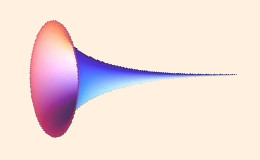It is topologically T2 ⊗ [0,∞), has finite surface area (2π) and (interior) volume (π/3), and constant negative curvature; the hyperbolic radian is taken to be unit distance. Note that the surface is not extensible at the t=0 end; the tangent with respect to t is infinite there. The limit surface of the bounding torus is a horosphere (isometric to R2).

For a nonorientable cusp, substitute a Klein bottle for the T2. (Ratcliffe, p. 445)

(Thurston Notes, p. 38, 116, 129, 139) (Thurston How..., p. 2559, 2563)

The first integral betti number of a hyperbolic 3-manifold with cusps must be ≥ the number of cusps. (Callahan et al., p. 329)

Topological invariants of a cusped manifold include:

• the volume of the largest cusp;
• the length of the shortest parabolic translation in the boundary of the maximal cusp;
• the ratio of the complex translations corresponding to the lifts of the meridian and longitude of the link component associated with a cusp: the homological cusp shape;
• the ratio of the lengths of the two shortest linearly independent (complex) parabolic isometric translations: the geometrical cusp shape.

The Chern-Simons invariant is defined modulo 1/2 for orientable manifolds. It changes sign with a change in orientation, so if there is an orientation reversing homomorphism of the manifold, the Chern-Simons invariant must be 0 or 1/4 mod 1/2.

(Callahan et al., p. 329)

• Any complete hyperbolic 3-manifold M is the quotient of H3 by a Kleinian group G, with π1(M) isomorphic to G.
A Kleinian group is a discrete torsion-free group of isometries of H3 (and is therefore a discrete subgroup of Moeb(E2)), which acts properly discontinuously (the inverse image of any compact set is also compact). The latter implies that a discrete group has a countable number of elements. Kleinian groups are usually taken to be nonelementary - they leave an infinite number of fixed points on the sphere at ∞ (the dimension of the limit set dim(Λ) > 0).

Λ(G) of a Kleinian group in H3 is on the sphere at ∞, and the regular set is tiled by solids whose vertices meet Λ(G) at ∞ (the surface tiles we discussed above are the faces of the solid tiles where they meet the sphere at ∞). If the solid tiles of a Kleinian group only touch the sphere at ∞ at ideal vertices, Λ(G) is the entire sphere. The figure 8 knot complement is an example of such a group; the solid tiles have infinitely many sides. (Mumford et. al., ch. 12)

∂M(G) = Ω(G) / G, and is the union of a finite number of hyperbolic Riemann surfaces with at most a finite number of punctures (arising from parabolic fixed points) and conical points (arising from elliptic fixed points). If g is the genus of the surface, n is the number of punctures and ri is the order of the ith cone point (ri finite and ≥ 2), each boundary component is constrained by
2 g + n - 2 + Σ (1 - 1 / ri) > 0 (see area formula above).
If G has no elliptic elements (as we will usually assume), 3g + n -3 ≥ 1, and the deformation (Teichmuller) space has complex dimension 3g + n - 3. The boundary surfaces have finite area 2π (2g + n - 2) > 0, so the boundary components can be:

• a sphere with 3 or more punctures,
• a torus with at least 1 puncture, or
• any higher-genus Riemann surface with zero or more punctures.

If G has N generators, Σ gi ≤ N. If G is purely loxodromic, ∂M(G) has ≤ N/2 components.

Every closed geodesic in M(G) is the projection of a loxodromic axis (connecting the fixed points of the loxodromic element) which is independent of any elliptic elements of G.

The fixed point of a parabolic map is on the sphere at ∞. There are an infinite number of horospheres tangent to the fixed point which are left invariant by the map. A horoball is the union of a horosphere with its interior.

The projections of the universal horoballs of the group G into M(G) are mutually disjoint; there is one for each parabolic fixed point.
If the fixed point is not also fixed by an elliptic map, its stablizer is either cyclic or abelian with rank 2. If cyclic, the quotient of H3 by the stablizer is homeomorphic to a solid cusp cylinder (a solid infinitely long cylinder with its axis removed). If rank 2, the quotient is homeomorphic to a solid cusp torus (a solid torus with its core curve removed, having finite volume and surface area). The boundary of either surface is incompressible.
π1 of the cusp torus injects into π1(M).

The process of Dehn-filling a cusp of a finite-volume hyperbolic manifold described above follows the removal of the interior of a solid cusp torus. The removal gives M a torus boundary component but does not change π1(M).

Think of the construction of the figure 8 knot complement; the removal of the vertex (necessary to make the object a manifold) removes the core curve of the torus, making it a solid cusp torus. The knot (in general, link) is the removed axis of the solid cusp torus (in general, tori).

A solid pairing tube is a finite cylinder with its axis removed, joining small discs around two punctures associated with a rank one cusp. (Think of a pairing tube as a "pinch" joining two ε-thick parts of M, with the pinch stretched out slightly so that each puncture is on one thick part.)

The (mutually disjoint) ε-thin parts of M are disjoint from the universal horoballs and are either

• tubular neighborhoods of short geodesics,
• solid cusp cylinders associated with rank 1 cusps or
• solid cusp tori associated with rank 2 cusps.

M is geometrically finite iff it is compact except for a finite number of cusps, and any rank 1 cusps correspond to pairs of punctures, each determining a solid pairing tube.

If the volume of M is finite, Λ(G) is S2, ∂M is empty, there are no rank 1 cusps and at most a finite number of rank 2 cusps.

• If M is geometrically finite, 0 < dim(Λ) < 2.
• If Λ is an S1, dim(Λ) = 1.
• If Λ is a quasicircle, dim(Λ) > 1.
• If Λ is totally disconnected, dim(Λ) < 1.
• For a singly degenerate G (which is geometrically infinite), dim(Λ) = 2.

(Series, p. 1, 2, 5, 7) (Marden, sections 2.2, 2.3, 3.1 - 3.4, 3.6, problem 3.20, 4.9, 4.10)

• A Geodesic Lamination Λ is a subset of H2 containing a closed set of mutually disjoint geodesics. Each component is called a leaf; two leaves may have a common endpoint on ∂H2, but no leaf can end at a puncture. The components of H2 - Λ are called gaps and are ideal polygons; if there are no punctures, the gaps are ideal triangles.

The projection of a measured lamination Λ to R = H2 / G is a set of simple closed geodesics. The space formed by projecting out scalar multiplication is homeomorphic to S6g+2n-7 (for n punctures). That projective space is the boundary of the Teichmuller space of R; the resulting closure is homeomorphic to B6g+2n-6.

(Marden, sections 3.9, 5.11)

• If E2 is the sphere at ∞ of H3, the convex hull boundary of G is the smallest convex set in H3 which meets ∞ in Λ(G). It is the union of hemispheres whose (B2) boundaries contain Ω(G) and intersect Λ(G) in at least 3 points. (Mumford et. al., ch. 12)

The quotient of the convex hull by G is called the convex core of M(G). It is a subset of the interior of M. Every closed geodesic in M(G) lies in the convex core (the convex core is the smallest convex set containing all closed geodesics of M(G)).

The boundary components of the convex core are incompressible iff Λ(G) is connected.
The convex core has finite volume iff G is geometrically finite. If G is geometrically finite without rank 1 cusps, all rank two cusps have solid cusp tori contained in the convex core.

(Marden, section 3.11)

• The compact core of a hyperbolic manifold M(G) with G finitely generated is a compact, connected submanifold of the interior of M(G) whose fundamental group is isomorphic to π1(M(G)) and whose boundary components are the full boundaries of noncompact components of the interior of M(G). For a given M(G), all compact cores are isomorphic.
M(G) can be separated into a "non-cuspidal part" Mnc(G) and a finite set of solid cusp cylinders and solid cusp tori. The ∂Mnc(G) is either a component of ∂M(G) or a doubly infinite cusp cylinder or a cusp torus.

The relative compact core Crel is defined similarly to the compact core, but has additional boundary components: a closed incompressible annulus which intersects each solid cusp cylinder, and the (also incompressible) cusp tori.

π1(Crel) is isomorphic to π1(M).
(Marden, section 3.12)
• Mostow rigidity implies that if hyperbolic manifolds of finite volume (n ≥ 3) M(G) and M(H) are diffeomorphic, then there is an isomorphism relating G and H. (Marden, section 3.13)
• An end of M(G) corresponds to an ideal boundary component (on the sphere at ∞) of the compact core of M(G). A relative end of M(G) corresponds to a non-annulus boundary component of the relative compact core of M(G). Thus M can be decomposed into a compact core and a set of ends.

And end of M(G) (or Mnc) is geometrically finite if it has a neighborhood which does not intersect the convex core of M(G). Otherwise, it is geometrically infinite, and the convex core extends into the end.

(Marden, section 5.5)

• All hyperbolic manifolds M(G) (with G finitely generated) are tame: the interior of M(G) is homeomorphic to the interior of a compact manifold, and every (relative) end has a neighborhood V which is homeomorphic to S ⊗ [0,∞) where S is ∂V (S is essentially the shape of the end at ∞). There are no simple loops in ∂M(G) which bound an essential disc in the interior of M(G). (Marden, section 5.6)
• An ending lamination is a (unique) geodesic lamination of S whose geodesics "exit" the (∞) end. Every simple closed geodesic in S crosses the lamination. Any geometrically infinite end is completely characterized by its ending lamination. (Marden, section 5.7)
• The hyperbolic Laplace-Beltrami operator in the unit ball model is
Δhu = ((1 - r2)2 / 4) (Δu + (2r/(1 - r2)) ∂u/∂r,
where Δu is the Euclidean laplacian.

If G is nonelementary and M(G) has infinite volume,

• λ0 = 0 and d = 2 iff G is geometrically infinite,
• λ0 = 1 iff G is geometrically finite and d ≤ 1, and
• 0 < λ0 = d(2-d) < 1 iff G is geometrically finite and 1 < d < 2,

where λ0 is the lowest eigenvalue of the hyperbolic Laplace-Beltrami operator on M(G) and d is the Hausdorff dimension of Λ(G) of G. (Marden, section 5.15)

• To summarize:

• A hyperbolic manifold M can be constructed as the quotient of H3 by a Kleinian group G (which is discrete, and we will assume it has no elliptic elements). Its limit set Λ(G) lies on the S2 at ∞.
• If G has n generators and no parabolic elements, Λ(G) is totally disconnected, and the quotient ∂M(G) of its regular set Ω(G) by G is a Riemann surface of genus n (possibly with twists).
• Such a surface has 3g-3 loops (which divide ∂M(G) into 2g-2 pairs of pants) which can be pinched by parabolic elements in G. Each parabolic element generates a cusp puncture in ∂M(G) corresponding to a solid pairing tube.
• When Λ(G) is a quasicircle, for each additional equivalence class of parabolic elements, one component of Ω(G) will break up into an infinite set of tangent discs.
• Non-degenerate groups are geometrically finite.
• Each degenerate component of Ω(G) has zero area (it is filled by Λ(G), which has dimension 2).
• If G is maximally degenerate, all of Ω(G) has zero area and Λ(G) is the S2 at ∞.
• Each cusp in a degenerate group corresponds to either a solid cusp cylinder (for a rank 1 cusp, which makes the volume of M infinite), or a solid cusp torus (for a rank 2 cusp, which makes M geometrically infinite but with finite volume).
• Each solid pairing tube is characterized by a (homotopy) winding number.
• Each solid cusp torus is characterized by a pair of winding numbers (for each homotopy generator of the torus).
• Each degenerate end of M(G) corresponding to a solid cusp cylinder is characterized by its ending lamination, which can be thought of as the irrational winding number of the infinite end.
• π1 of each cusp injects into π1(M(G)).
• Further manifolds can be created by Dehn filling solid cusp tori.

#### Orbifolds

An orbifold is a Hausdorff space M which is the quotient of a geometric space X by a discrete subgroup Γ of the similarities of X which do not necessarily act freely (Γ contains elliptic elements). In particular:

• If a cone angle is irrational, Γ is not discrete and the object is then called a cone manifold.
• Tha analog of the covering map is the developing map, which "unrolls" the orbifold in H3. The corresponding holonomy map sends π1((M - cone axes) / elliptical elements) to the group of Moebius transformations generated by the elliptical elements.
• If two cone manifolds are homeomorphic with corresponding cone angles equal (and at most π), the two manifolds are isometric.
(
Marden, section 4.11)

• A singular point is a point whose stabilizer is of order > 1.
• If X is Sn, Rn or Hn, X / Γ and X / Κ are isometric iff Γ and Κ are conjugate in the isometry group of X.
• The link of a cusp point of a complete hyperbolic 3-orbifold has trivial holonomy.
• Dehn surgery invariants of a hyperbolic 3-orbifold need not be relatively prime (d is the greatest common divisor).
• A complete 3-orbifold is homeomorphic to a manifold obtained from (p/d, q/d) Dehn surgery on a knot complement.
(Ratcliffe, ch. 13)

• A 3-orbifold is topologically a manifold; higher dimensional orbifolds are not necessarily manifolds.
(Abikoff, section 1)

## On Knots

If you're going to play with knots, I recommend building a jig; tying knots without something to hold the crossovers in place can be very difficult. I simply cut a square piece of laminate and hammered 25 nails into it. Knot 63 (from the
standard tables) is shown here: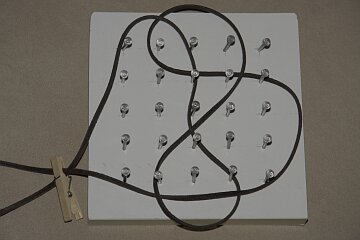• S1 is the unknot.

The crossing number of a knot is the minimum number of crossings for all planar projections of the knot. It is an isotopy invariant.

An isotopy is a homotopy between embeddings (making it a homeomorphism).
For an oriented link, we can assign a value to each crossing of different components:
• +1 if rotating the overstrand in a counterclockwise direction will align it with the understrand;
• -1 if rotating the overstrand in a clockwise direction will align it with the understrand.
The linking number is half of the sum of those crossing numbers, and is also an isotopy invariant.

For a projection of an oriented link, the writhe is the sum of the crossing numbers for all crossings. It is not an isotopy invariant.

A knot which can be separated into nontrivial sub-knots, each of which can be enclosed in a sphere intersected by only two arcs, is composite (denoted as K1 # K2). If such a decomposition is not possible, the knot is prime.

The number of prime knots with each crossing number up to 15 have been computed:
 3 4 5 6 7 8 9 10 11 12 13 14 15 1 1 2 3 7 21 49 165 552 2176 9988 46972 253293
A knot which is isotopically equivalent to its mirror image is amphicheiral.
Of the knots up to 9 crossings, the only achiral knots are 31, 63, 83, 89, 812, 817 and 818.
A sub-knot whose planar projection intersects a surrounding circle four times is a tangle.

A knot formed by rotation or inversion of a tangle is a mutant.

An alternating knot is a knot whose crossing signs alternate as the knot is traversed in a given direction.

A knot which can be invertibly mapped to the surface of a torus is a torus knot.

A (p,q)-torus knot wraps around the meridian p times and around the longitude q times. A (9,2) torus knot: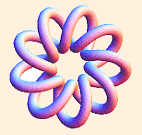If a knot is embedded in an unknotted solid torus, and that solid torus is then knotted, the resultant embedded knot is a satellite knot. (Adams, pp. 2-3, 8-9, 15, 19, 41, 49, 108, 115, 152) (Lickorish, p. 6)

• If K1 # K2 is an alternating knot, their crossing numbers are simply additive. (Adams, p. 69)
• A (p,q)-torus knot is also a (q,p)-torus knot.

The crossing number for a (p,q)-torus knot is min (p (q - 1), q (p - 1)). (Adams, pp. 110-111)

• A (p,q)-torus knot can be parametrized on a torus of radius 1/4 by

• x(t) = cos (p t) + cos (p t) cos (q t ) / 4
• y(t) = sin (p t) + sin ( p t) cos ( q t) / 4
• z(t) = sin ( q t) / 4

There are similar expansions for Lissajous knots and the knots 31, 41, 51 and 819. (Kauffman (Fourier), p. 366) (Trautwein, pp. 355-356, 359, 361)

• Torus knots and satellite knots are not hyperbolic. All other knots are.
A hyperbolic knot is a knot whose complement can be given a metric of constant curvature -1.